Homework Help Question & Answers

# 8. If ū= 8î - 159 and v = -3i - 4ſ and w = 12...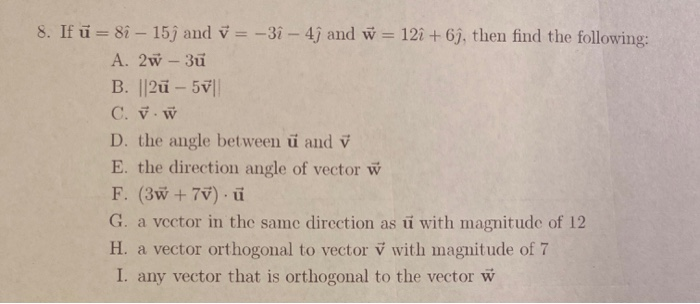8. If ū= 8î - 159 and v = -3i - 4ſ and w = 12 + 69, then find the following: A. 2w - 3ū B. ||2u - 57 C. v. W D. the angle between ü and v E. the direction angle of vector w F. (3 +70).ü G. a vector in the same direction as ū with magnitude of 12 H. a vector orthogonal to vector v with magnitude of 7 I. any vector that is orthogonal to the vector w

#### Homework Answers

Answer #1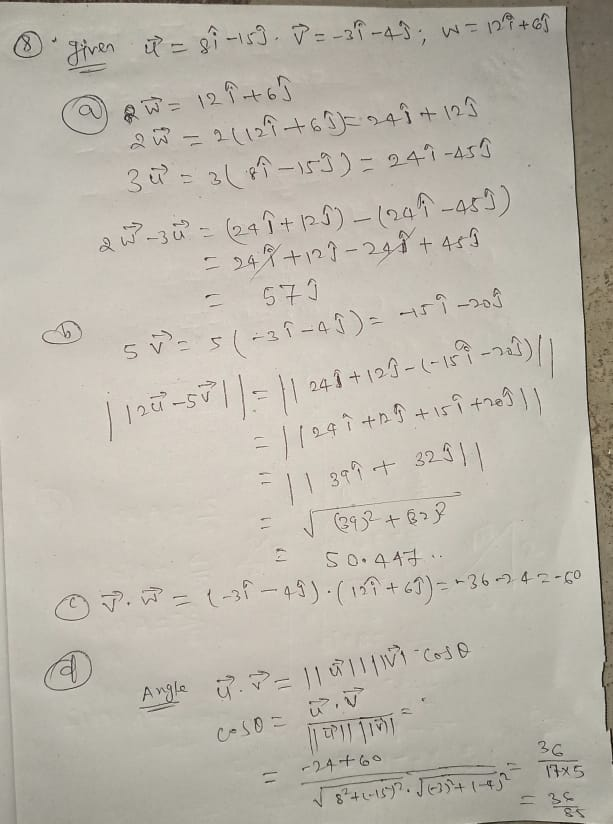Know the answer?
Your Answer:

#### Post as a guest

Your Name:

What's your source?

#### Earn Coin

Coins can be redeemed for fabulous gifts.

Not the answer you're looking for? Ask your own homework help question. Our experts will answer your question WITHIN MINUTES for Free.
Similar Homework Help Questions
• ### 8. If ü= 8i - 15j and = -31 - 4; and w = 121 +...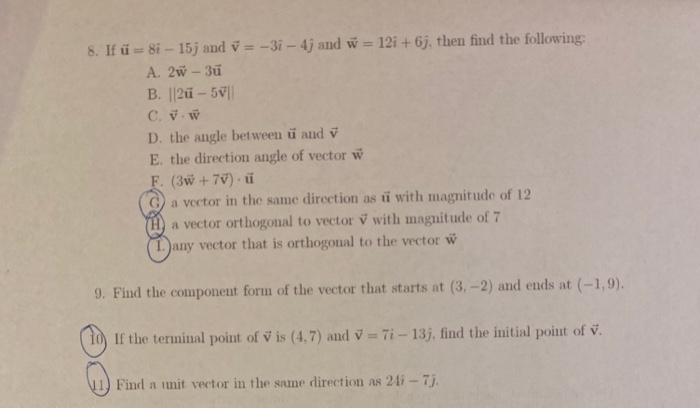8. If ü= 8i - 15j and = -31 - 4; and w = 121 + 6j, then find the following: A. 2w - 3ü B. ||2u - 501 C. J. D. the angle between ü and v E. the direction angle of vector w F. (3x + 70). a vector in the same direction as u with magnitude of 12 a vector orthogonal to vector V with magnitude of 7 any vector that is orthogonal to the vector w...

• ### Given vectors ü = (-1,5), i = 3i – 4j, w = (2,7), find: (2pts each)...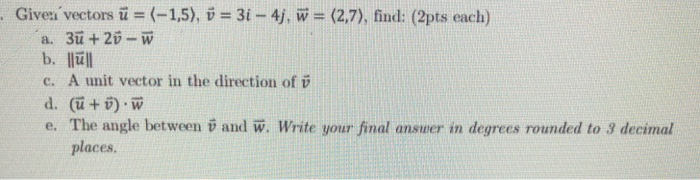Given vectors ü = (-1,5), i = 3i – 4j, w = (2,7), find: (2pts each) a. 3ū + 20 - w b. llull c. A unit vector in the direction of v d. (ü + ). W e. The angle between ï and W. Write your final answer in degrees rounded to 3 decimal places.

• ### I need help with question 2b and 3. Please help it would be awesome if i knew how to do these questions. v=(2,-4) w=-3i+...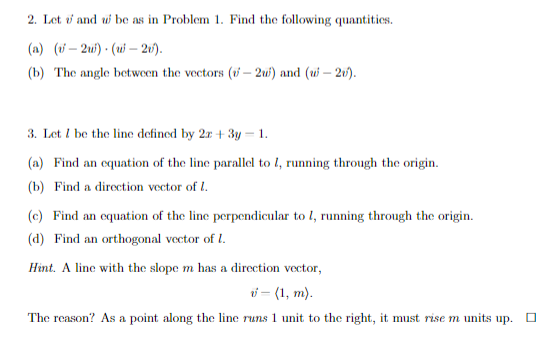I need help with question 2b and 3. Please help it would be awesome if i knew how to do these questions. v=(2,-4) w=-3i+2j 2. Let and ui be as in Problem 1. Find the following quantitics (a) 2u) (ui-2t). (b) The angle between the vectors (2u) and (wi-2) 3. Let be the line defined by 2r +3y 1. (a) Find an equation of the line parallel to I, running through the origin. (c) Find an equation of the line...

• ### how to do number 16 16) (6pts) Find the projection of ü onto w. a) Find ui --Son 347 2 25 26 b) Find 펄 orthogon...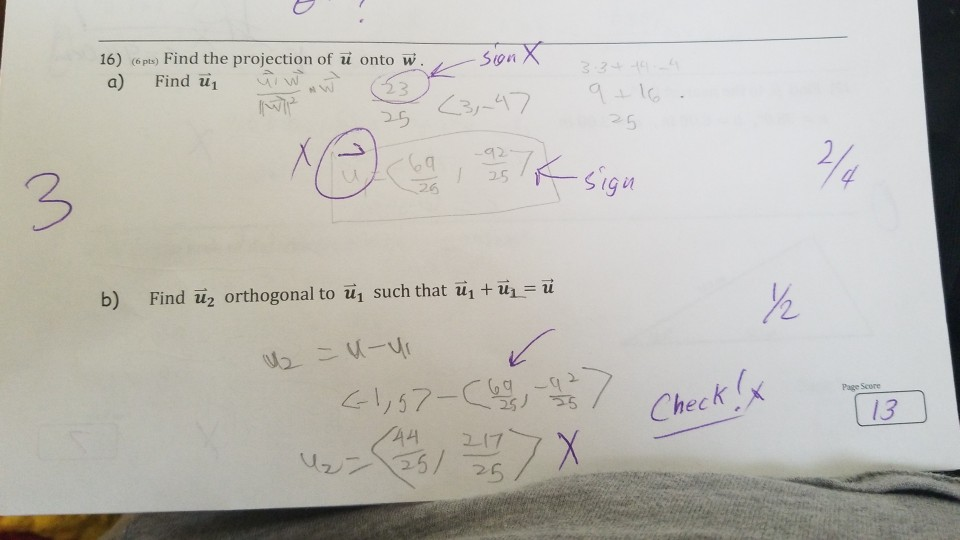how to do number 16 16) (6pts) Find the projection of ü onto w. a) Find ui --Son 347 2 25 26 b) Find 펄 orthogonal to in such that iiitül ü 2 Page Score Check ( 13 For problems on this page, use the vectors described graphically here. Your work should include correct vector notation of u, i,and w 13) What is (w+u) v E xplat 3 U=(2、1) w (3, .. 4) 14) Find the cxact magnitudes of i,...

• ### 9. Find the component form of the vector that starts at (3,-2) and ends at (-1,9)....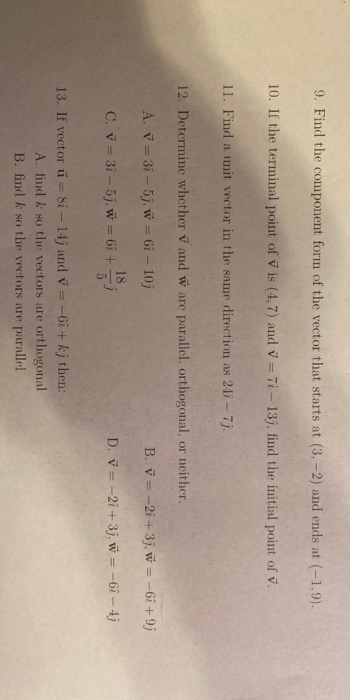9. Find the component form of the vector that starts at (3,-2) and ends at (-1,9). 10. If the terminal point of vis (4.7) and v = Ti - 13), find the initial point of v. 11. Find a imit vector in the same direction as 211 - 7. 12. Determine whether V and w are parallel. orthogonal, or neither. B. v= -2i+3j, w = -6i+9j A. V = 3i-57. w = 6i - 103 18 C. v = 3i...

• ### PLEASE SHOW WORK!!!!!! 16) On a man the town of Morgan Run is due south of...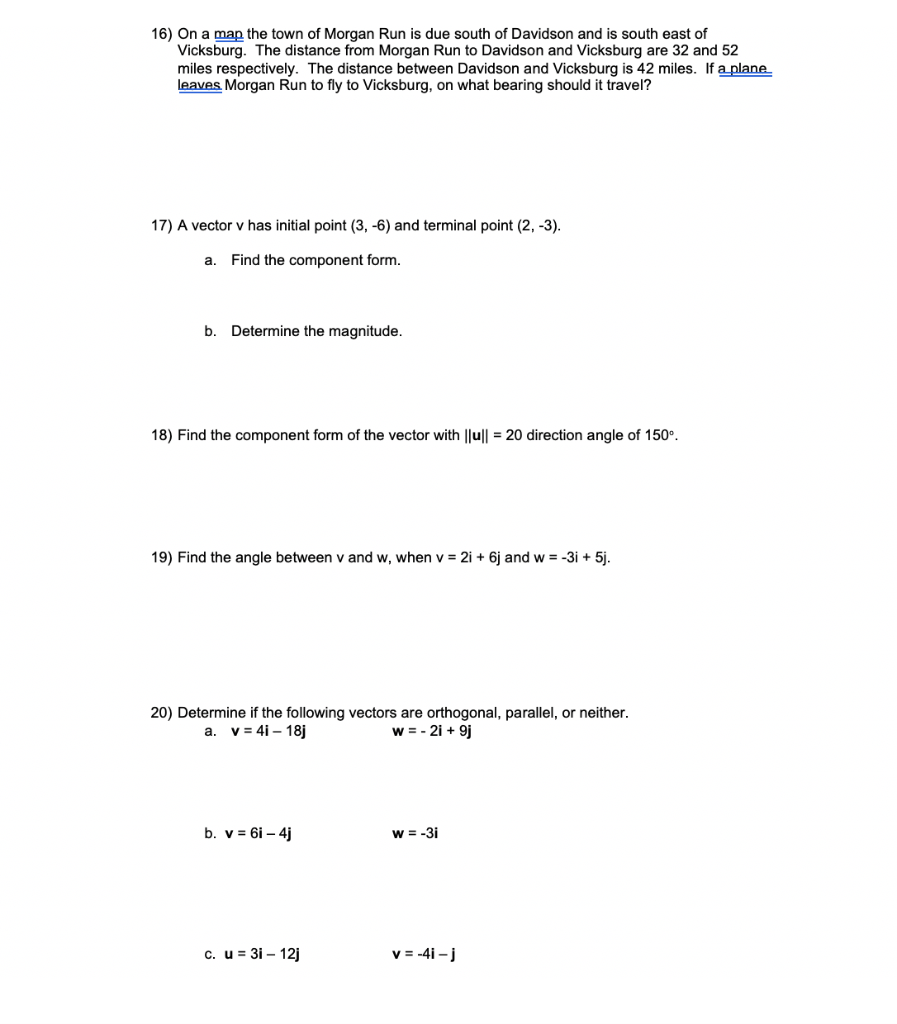PLEASE SHOW WORK!!!!!! 16) On a man the town of Morgan Run is due south of Davidson and is south east of Vicksburg. The distance from Morgan Run to Davidson and Vicksburg are 32 and 52 miles respectively. The distance between Davidson and Vicksburg is 42 miles. If a plane leaves Morgan Run to fly to Vicksburg, on what bearing should it travel? 17) A vector v has initial point (3,-6) and terminal point (2, -3). a. Find the component...

• ### 6-7. Given vectors U = -4i +12, V = 5i - 2j, W=-3i- 6. Find a)...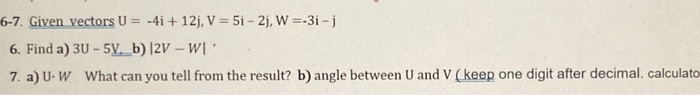6-7. Given vectors U = -4i +12, V = 5i - 2j, W=-3i- 6. Find a) 30 - 5V._b) 2V - W'. 7. a) UW What can you tell from the result? b) angle between U and V (keep one digit after decimal. calculato

• ### problem 1 and problem 2 please , thankyou very much PROBLEM 1 (25%) Find: Let: ū=...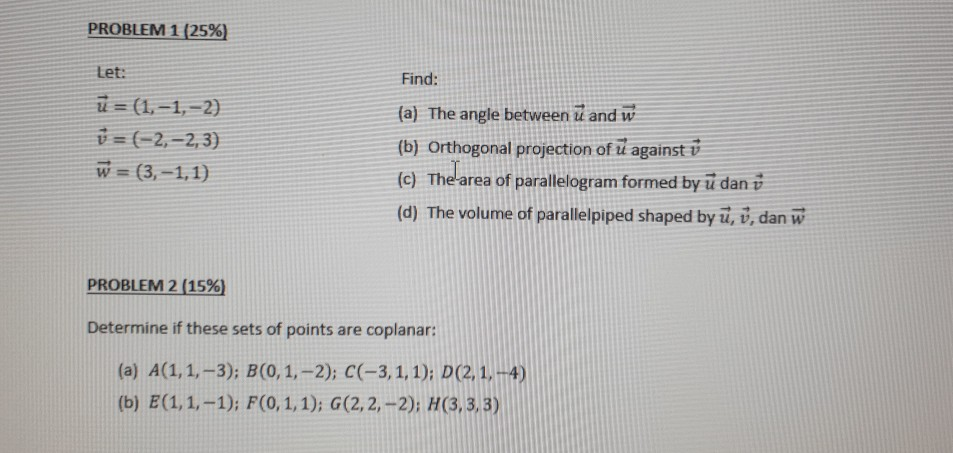problem 1 and problem 2 please , thankyou very much PROBLEM 1 (25%) Find: Let: ū= (1,-1,-2) v = (-2,-2,3) w = (3,-1,1) (a) The angle between ū and w (b) Orthogonal projection of u against v (c) The area of parallelogram formed by u dan v (d) The volume of parallelpiped shaped byū, v, dan w PROBLEM 2 (15%) Determine if these sets of points are coplanar: (a) A(1,1,-3); B(0,1,-2); C(-3, 1, 1); D(2, 1, -4) (b) E(1,1,-1); F(0,1,1);...

• ###  Given: u = -81 + 6j v = 1-3 w = 41 - 8j T=...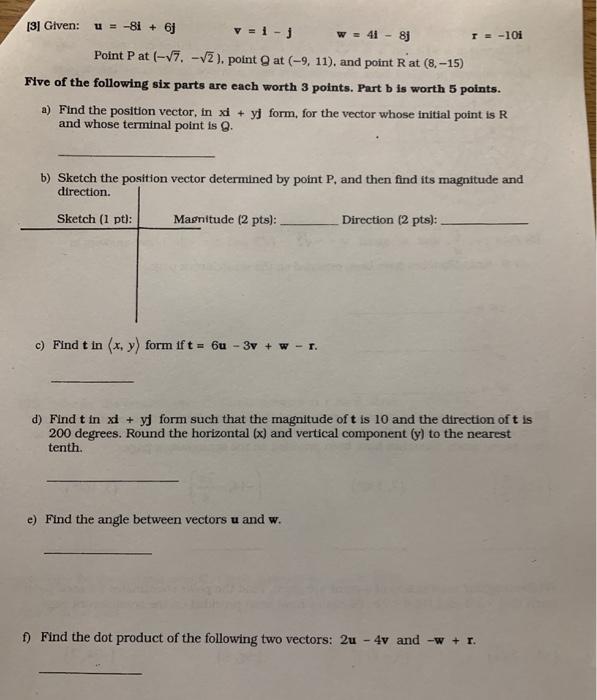Given: u = -81 + 6j v = 1-3 w = 41 - 8j T= -101 Point Pat (-7, -2), point at (-9, 11), and point Rat (8.-15) Five of the following six parts are each worth 3 points. Part b is worth 5 points. a) Find the position vector, in x + yj form, for the vector whose initial point is R and whose terminal point is g. b) Sketch the position vector determined by point P, and...

• ###  Given: u = -8i + 6 v = i- j w = 4i - 8j...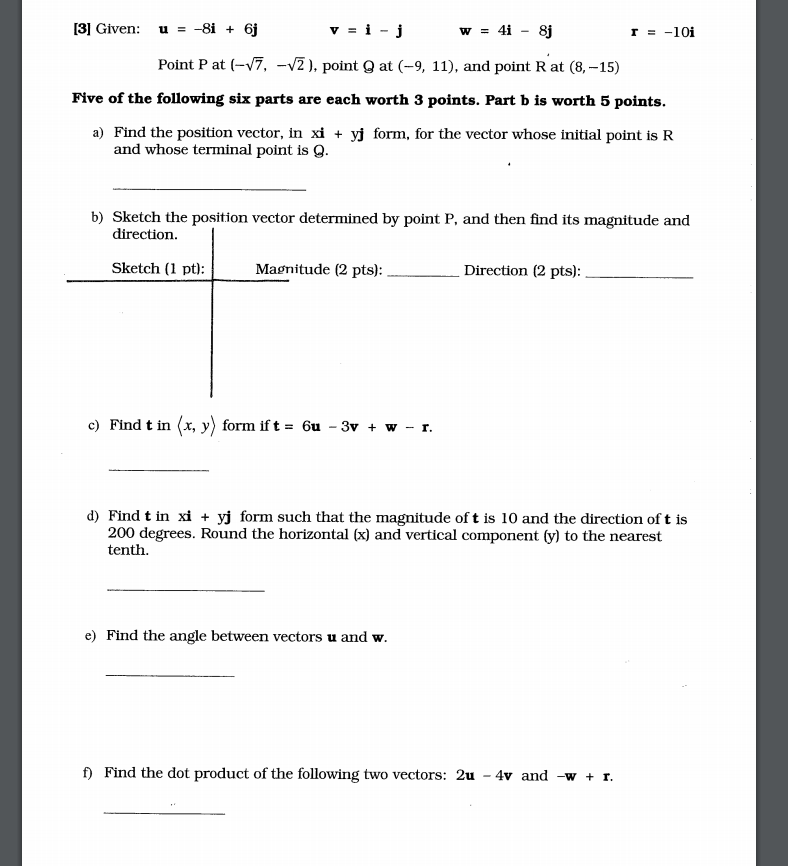Given: u = -8i + 6 v = i- j w = 4i - 8j T = -10i Point Pat (-17, -v2), point at (-9, 11), and point Rat (8, -15) Five of the following six parts are each worth 3 points. Part b is worth 5 points. a) Find the position vector, in xi + yj form, for the vector whose initial point is R and whose terminal point is 9. b) Sketch the position vector determined by...

Free Homework App

Scan Your Homework
to Get Instant Free Answers
Need Online Homework Help?

Get Answers For Free
Most questions answered within 3 hours.